Github: msight-tech/research-ms-loss

## 1. MS Loss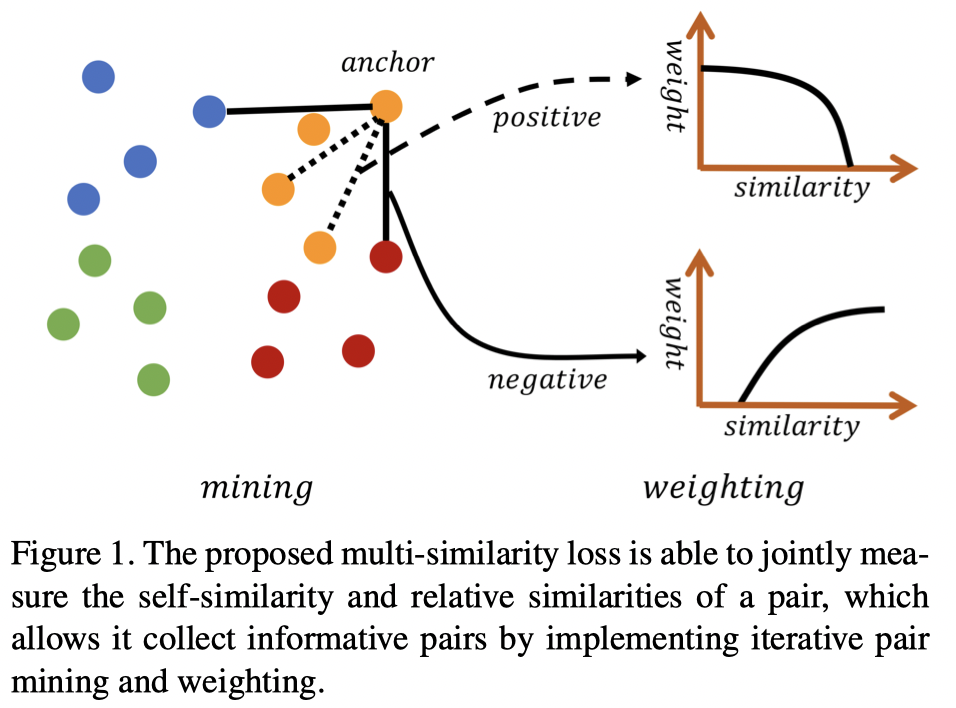MS Loss 定义：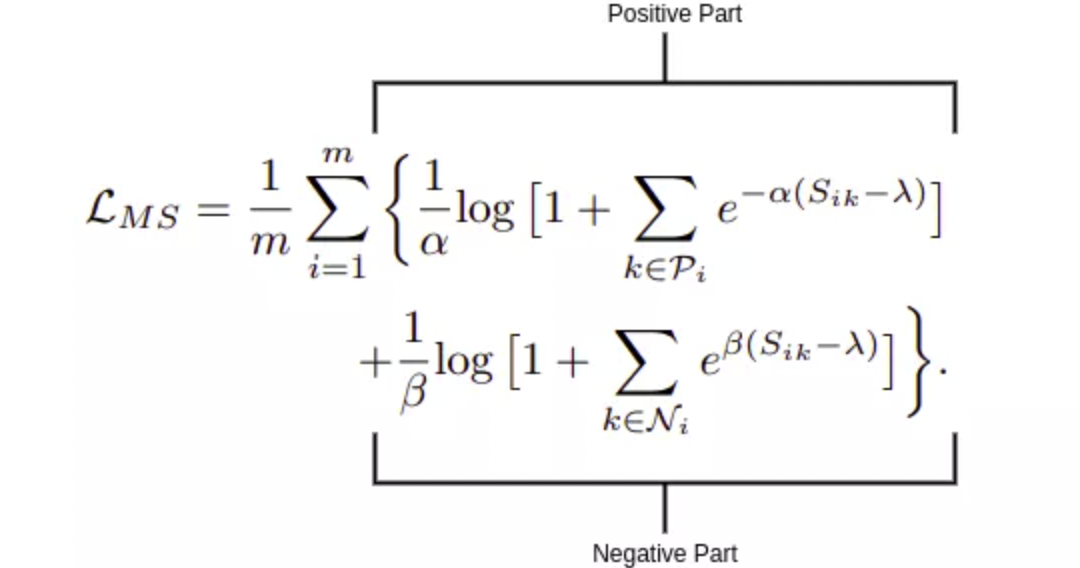MS Loss 包含两部分：正样本部分和负样本部分.

### 1.1. 正样本部分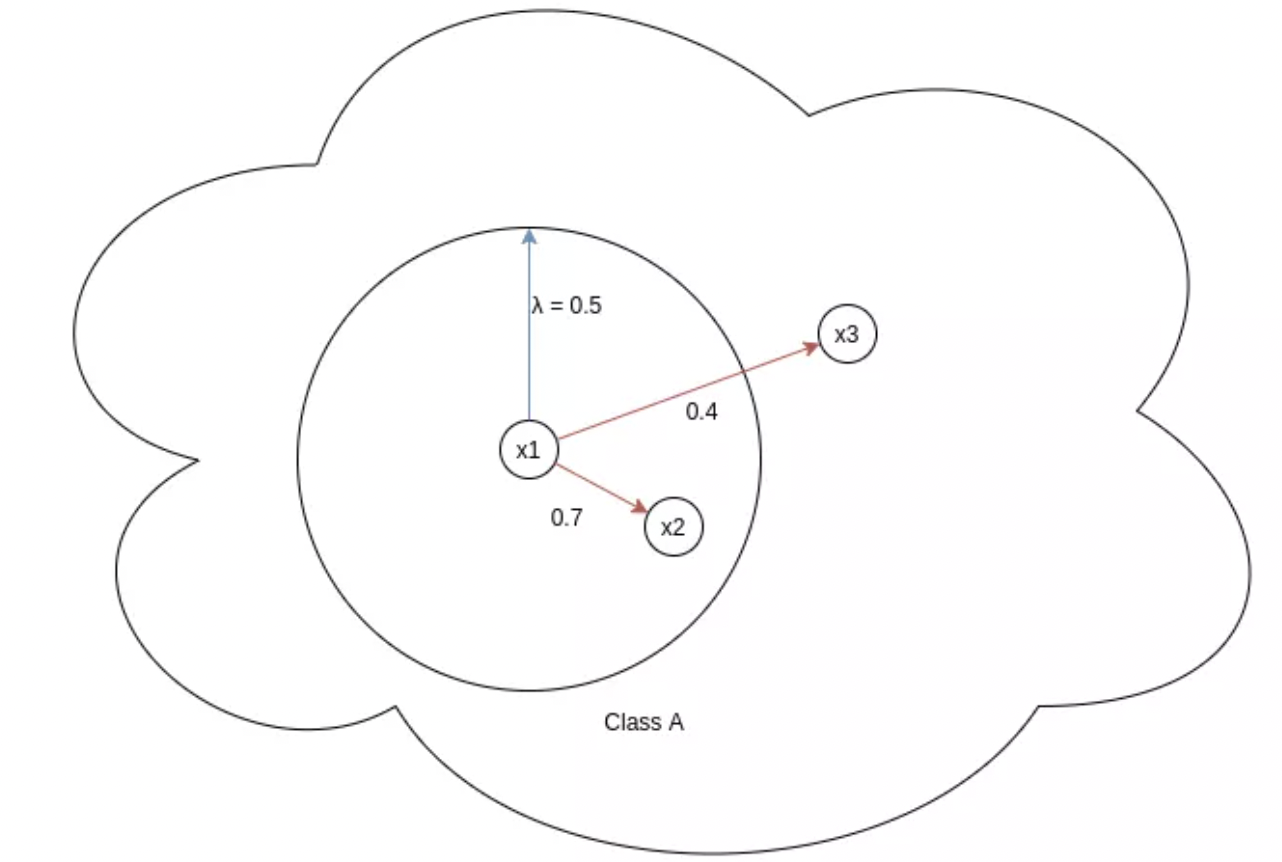### 1.2. 负样本部分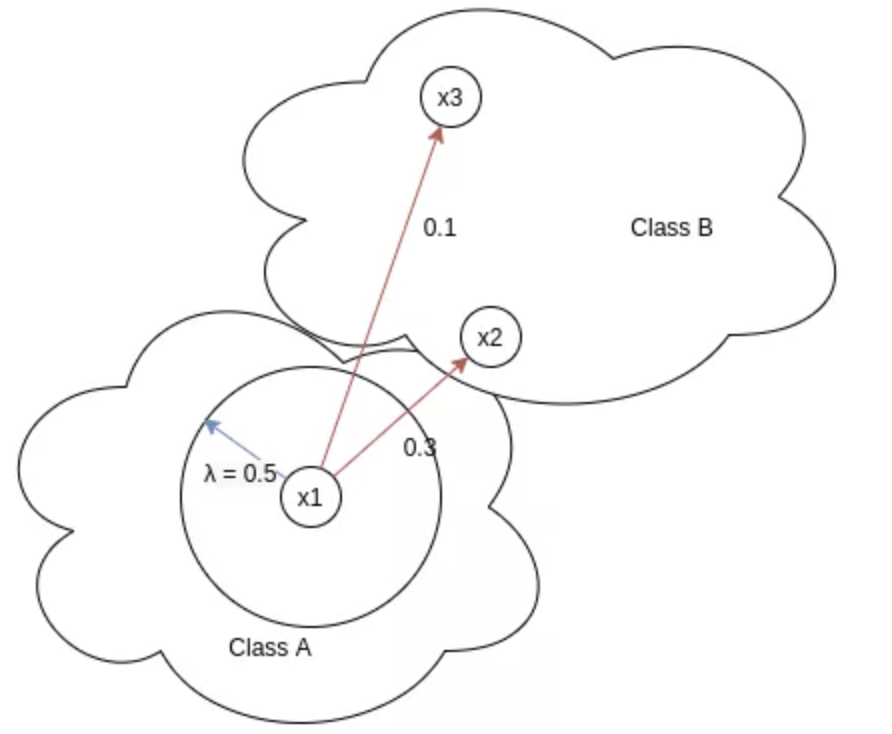## 2. 样本对相似性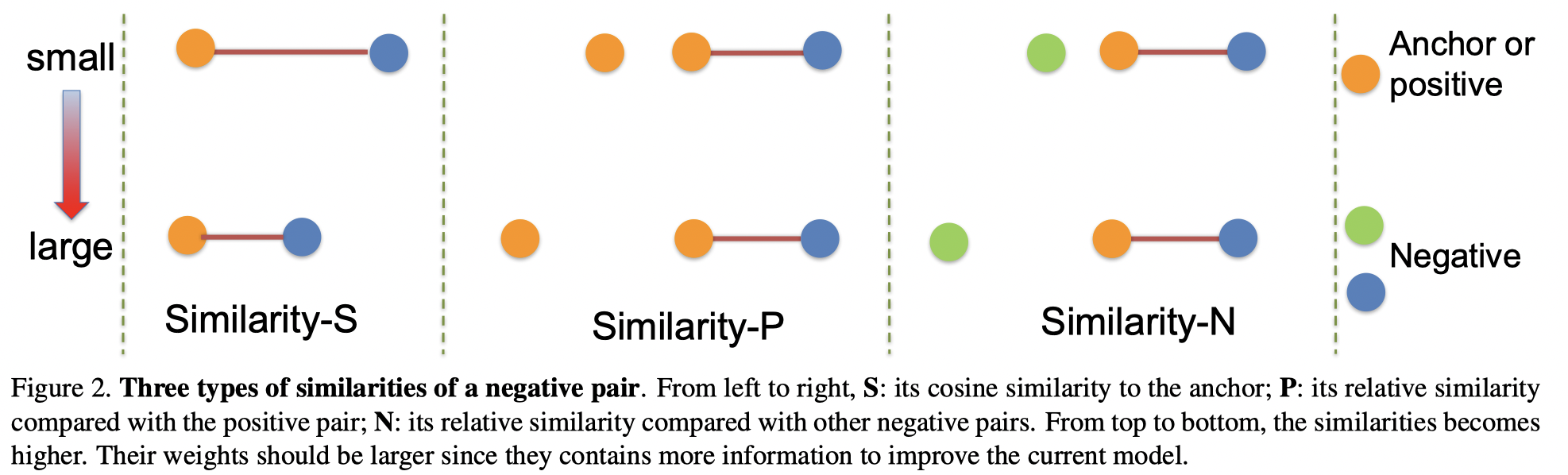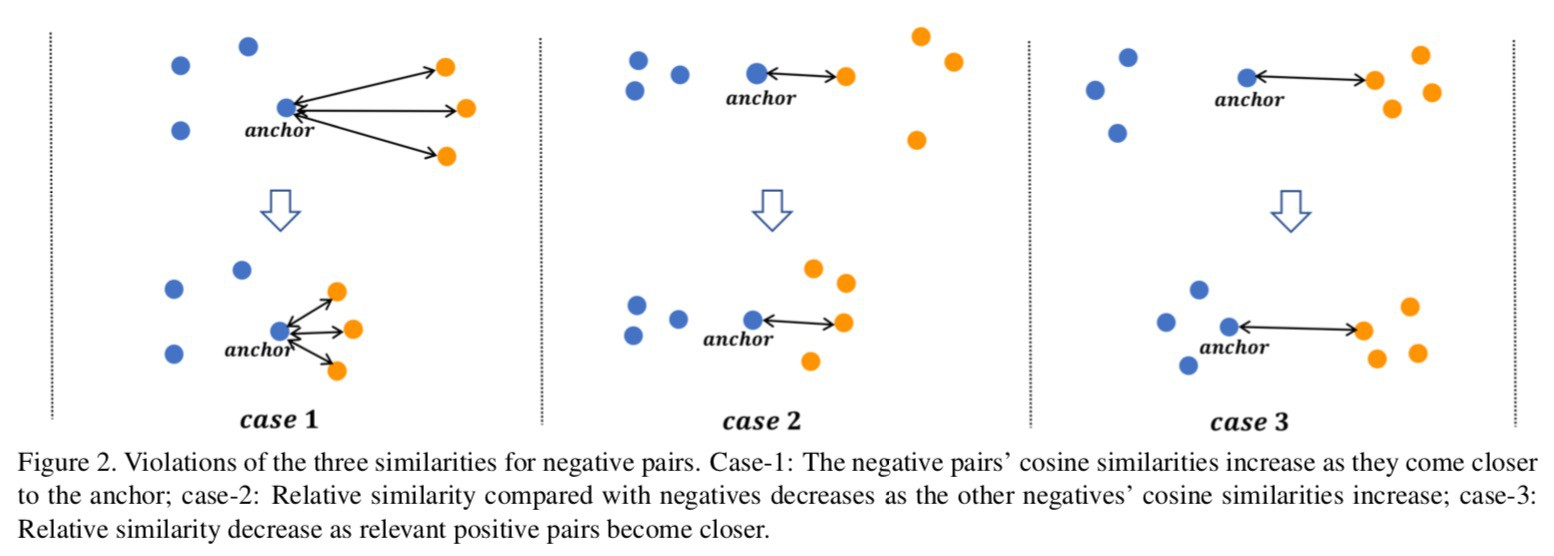- 自相似性：根据样本对自身计算出的相似性，这是一种最常用也是最重要的相似性. 例如，当一个负样本对的余弦相似性较大时，意味着很难把该样本对所对应的两种类别区分开来，这样的样本对对模型来说是困难的，也是有信息量的，对于模型学习更有区分度的特征很有帮助. 另一方面，自相似性很难完整地描述embedding空间的样本分布情况.

 - 正相对相似性：不仅考虑当前样本对自身的相似性，还考虑局部邻域内正样本对之间的相对关系.

 - 负相对相似性：不仅考虑当前样本对自身的相似性，还考虑局部邻域内负样本对之间的相对关系.

### 2.1. 自相似性(Similarity-S)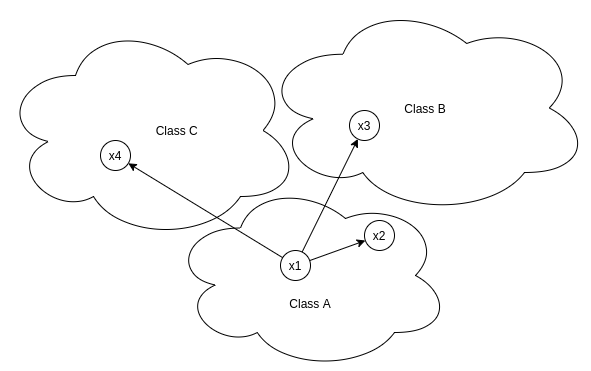### 2.2. 负样本相对相似性(Similarity-N)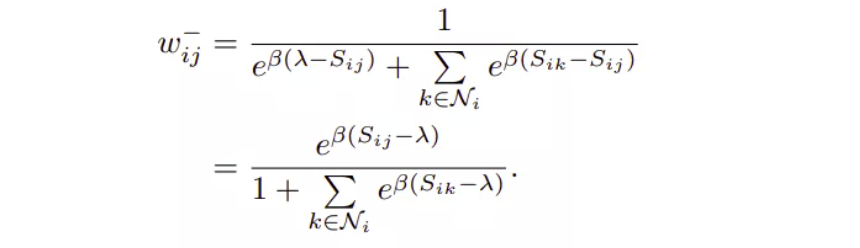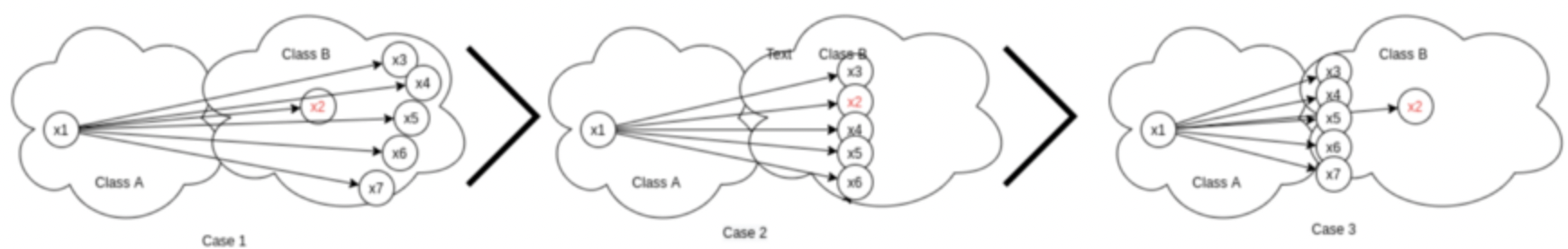- Case1 - 所有其他负样本相对于 x1 的距离比 x2 与 x1 的距离更远.

 - Case2 - 所有其他负样本相对于 x1 的距离和 x2 与 x1 的距离一样.

 - Case3 - 所有其他负样本相对于 x1 的距离比 x2 与 x1 的距离更近.

 - Case1 - $w_{ij}$ 最大，因为 $\sum e^{(\beta(S_{ik} - S_{ij}))}$ 最小，$S_{ik} < S_{ij}$，使得指数为负数.

 - Case2 - $w_{ij}$ 中等，因为 $\sum e^{(\beta(S_{ik} - S_{ij}))}$ 指数为 0.

 - Case3 - $w_{ij}$ 最小，因为 $\sum e^{(\beta(S_{ik} - S_{ij}))}$ 最大，$S_{ik} > S_{ij}$，使得指数为正数.

### 2.3. 正样本相对相似性(Similarity-P)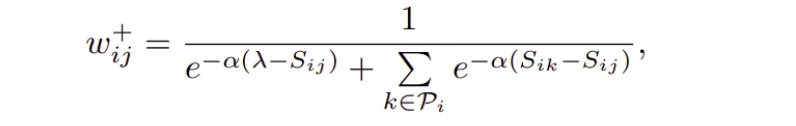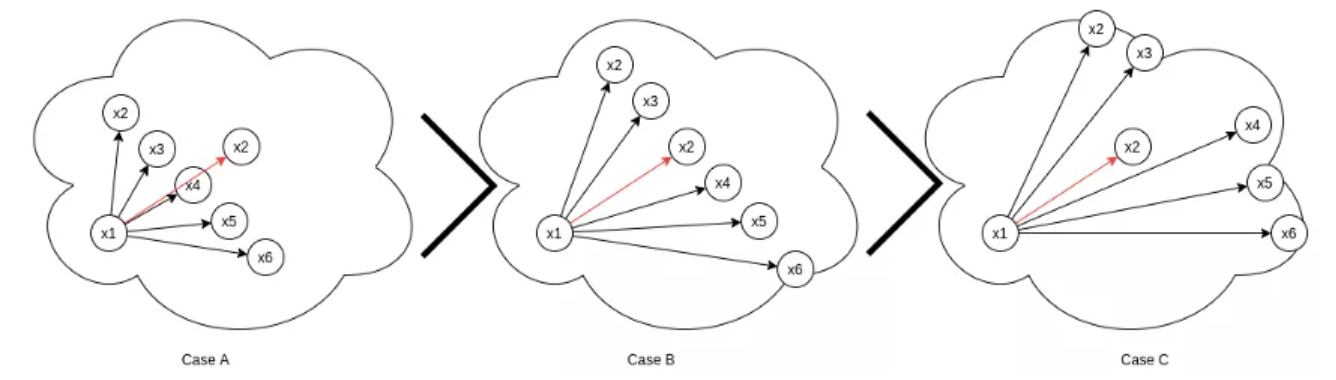### 2.4. 不同损失函数的相似性类型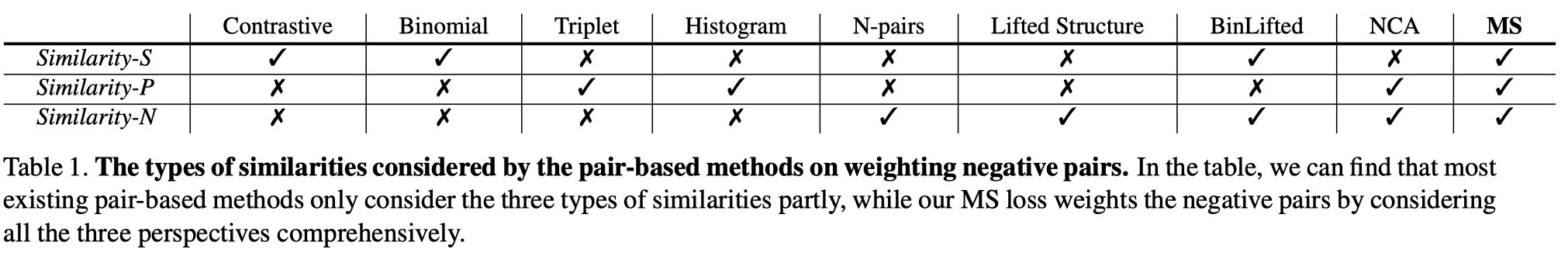## 3. 难样本挖掘

MS Loss 的作者在训练时仅使用了困难的负样本和正样本，并丢弃了其他的样本对，因为它们对效果提升几乎没有贡献，同时还降低了性能，只选择了那些具有更多价值信息的样本对，也使得算法计算速度更快.### 3.1. 难负样本挖掘

$$S_{ij}^{-} > \text{min}_{y_k = y_i} S_{ik} - \epsilon$$

### 3.2. 难正样本挖掘

$$S_{ij}^{+} < \text{min}_{y_k \neq y_i} S_{ik} + \epsilon$$

## 4. MS Loss 实现

research-ms-loss/multi_similarity_loss.py

import torch
from torch import nn

from ret_benchmark.losses.registry import LOSS

@LOSS.register('ms_loss')
class MultiSimilarityLoss(nn.Module):
def __init__(self, cfg):
super(MultiSimilarityLoss, self).__init__()
self.thresh = 0.5
self.margin = 0.1
#alpha
self.scale_pos = cfg.LOSSES.MULTI_SIMILARITY_LOSS.SCALE_POS
#beta
self.scale_neg = cfg.LOSSES.MULTI_SIMILARITY_LOSS.SCALE_NEG

def forward(self, feats, labels):
# feats: 图片的提取特征，l2 归一化的向量
# labels：图片的 GT 类别
assert feats.size(0) == labels.size(0), \
f"feats.size(0): {feats.size(0)} is not equal to labels.size(0): {labels.size(0)}"
batch_size = feats.size(0)
#相似性矩阵，l2归一化向量与其转置的点积
#sim_mat(i,j) - 行和列，对应于batch内第i个向量和第j个向量的相似性.
#sim_mat维度为 batch_size * batch_size.
#sim_mat的第0行对应于第0个向量与batch内其余向量的相似性.
sim_mat = torch.matmul(feats, torch.t(feats))

epsilon = 1e-5
loss = list()
for i in range(batch_size):
#第i个向量是 anchor.
#获取所有的正样本对，通过匹配与anchor具有相同类别标签的向量.
pos_pair_ = sim_mat[i][labels == labels[i]]
#移除样本对，通过计算anchor与其自身的相似性，如相似度为1的样本对.
pos_pair_ = pos_pair_[pos_pair_ < 1 - epsilon]
#获取所有的负样本对，通过匹配与anchor具有不同类别标签的向量.
neg_pair_ = sim_mat[i][labels != labels[i]]
#挖掘困难负样本，采用3.1所述方法.
#将值为0.1的margin加到负样本对相似性，以得到恰好位于边界的负样本.
neg_pair = neg_pair_[neg_pair_ + self.margin > min(pos_pair_)]
#挖掘困难正样本，采用3.2所述方法.
pos_pair = pos_pair_[pos_pair_ - self.margin < max(neg_pair_)]

if len(neg_pair) < 1 or len(pos_pair) < 1:
continue

#如果困难正样本和困难负样本都存在，则继续计算损失.
#加权
pos_loss = 1.0 / self.scale_pos * torch.log(
1 + torch.sum(torch.exp(-self.scale_pos * (pos_pair - self.thresh))))
neg_loss = 1.0 / self.scale_neg * torch.log(
1 + torch.sum(torch.exp(self.scale_neg * (neg_pair - self.thresh))))
#MS Loss公式
loss.append(pos_loss + neg_loss)

if len(loss) == 0:
#
loss = sum(loss) / batch_size
return loss

import torch
from pytorch_metric_learning import miners, losses

class MultiSimilarityLoss(torch.nn.Module):
def __init__(self, ):
super(MultiSimilarityLoss, self).__init__()
self.thresh = 0.5
self.epsilon = 0.1
self.scale_pos = 2
self.scale_neg = 50

self.miner = miners.MultiSimilarityMiner(epsilon=self.epsilon)
self.loss_func = losses.MultiSimilarityLoss(self.scale_pos, self.scale_neg, self.thresh)

def forward(self, embeddings, labels):
#难样本挖掘
hard_pairs = self.miner(embeddings, labels)
#损失计算
loss = self.loss_func(embeddings, labels, hard_pairs)
return loss

## 5. 参考

Last modification：April 20th, 2021 at 06:23 pm